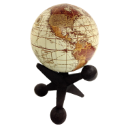# Convert lat,long to bbox using WGS-84

1129
9
11-21-2018 09:39 AMby
New Contributor II

I am stuck how to convert lat,long to bbox using WGS-84. Is there a formula or function code you could share.

e.g. lon=-79.80617638166511

lat=43.17565342984143

bbox?

``````
``````
1 Solution

Accepted SolutionsbyMVP Esteemed Contributor

Raju,

My code snippet above is for a map click function where the event object passed is the map click event.

Here is a function to convert a lat lon to an extent.

``````function onSelDrawEnd(lat, lon) {
var mapPnt = new Point(parseFloat(lon), parseFloat(lat));
var scrPnt = map.toScreen(mapPnt);
//Expand the point by 5 pixels
var xMin = scrPnt .x - 2.5; //value in pixels
var yMin = scrPnt .y - 2.5; //value in pixels
var xMax = scrPnt .x + 2.5; //value in pixels
var yMax = scrPnt .y + 2.5; //value in pixels
var mp1 = map.toMap(new Point(xMin, yMin));
var mp2 = map.toMap(new Point(xMax, yMax));
var ext = new Extent(mp1.x, mp1.y, mp2.x, mp2.y, new SpatialReference({ wkid:4326 }));
return Polygon.fromExtent(ext);
}‍‍‍‍‍‍‍‍‍‍‍‍‍‍‍‍‍‍‍‍‍‍‍‍‍‍``````
9 RepliesbyMVP Esteemed Contributor

Raju,

To get a bounding box from a single point you just need to do some math to add and subtract for that point to get the minx, miny, maxx and maxy for the extent class constructor.by
New Contributor II

Thanks Rob for always being helpful. I am using simple math to get bbox from lat,long. It is not accurate for closest zoom scale.

var minxx = lon - 0.08999;
var maxxx = lon + 0.08999;
var minyy = lat - 0.08999;
var maxyy = lat - 0.08999;

console.log("weblink/bbox?" + "xmin=" + minxx + "&ymin=" + minyy + "&xmax=" + maxxx + "&ymax=" + maxyy).

I have WGS-84 projection. Do I have to add condition for each scale. e.g.

If (scale =11)

var minxx = lon - 0.08999;
var maxxx = lon + 0.08999;
var minyy = lat - 0.08999;
var maxyy = lat - 0.08999;

elseif  (scale =19)

var minxx = lon - ??;
var maxxx = lon + ??;
var minyy = lat - ??;
var maxyy = lat - ??;byMVP Esteemed Contributor

Raju,

Normally I would be adding/subtracting values with a set number of pixels using toScreen and toMap.by
New Contributor II

Thanks Rob for your response. Could you kindly provide an example for set of pixels toScreen and toMap. I have a deadline to meet.byMVP Esteemed Contributor

You working in 3.x or 4.x?by
New Contributor IIbyMVP Esteemed Contributor

Raju,

Here is how I turn a point into a polygon to query by:

``````    function onSelDrawEnd(event) {
var geom = event.mapPoint;
//Expand the point by 5 pixels
var xMin = event.screenPoint.x - 2.5; //value in pixels
var yMin = event.screenPoint.y - 2.5; //value in pixels
var xMax = event.screenPoint.x + 2.5; //value in pixels
var yMax = event.screenPoint.y + 2.5; //value in pixels
var mp1 = map.toMap(new Point(xMin, yMin));
var mp2 = map.toMap(new Point(xMax, yMax));
var ext = new Extent(mp1.x, mp1.y, mp2.x, mp2.y, geom.spatialReference);
var pPoly = Polygon.fromExtent(ext);
queryFeaturesGraphical(pPoly);
}‍‍‍‍‍‍‍‍‍‍‍‍‍``````by
New Contributor II

Thanks Robert for sharing "turn point into a polygon".  Could you please guide how I can pass 'event' into my existing code (above code). Your help is appreciated.byMVP Esteemed Contributor

Raju,

My code snippet above is for a map click function where the event object passed is the map click event.

Here is a function to convert a lat lon to an extent.

``````function onSelDrawEnd(lat, lon) {
var mapPnt = new Point(parseFloat(lon), parseFloat(lat));
var scrPnt = map.toScreen(mapPnt);
//Expand the point by 5 pixels
var xMin = scrPnt .x - 2.5; //value in pixels
var yMin = scrPnt .y - 2.5; //value in pixels
var xMax = scrPnt .x + 2.5; //value in pixels
var yMax = scrPnt .y + 2.5; //value in pixels
var mp1 = map.toMap(new Point(xMin, yMin));
var mp2 = map.toMap(new Point(xMax, yMax));
var ext = new Extent(mp1.x, mp1.y, mp2.x, mp2.y, new SpatialReference({ wkid:4326 }));
return Polygon.fromExtent(ext);
}‍‍‍‍‍‍‍‍‍‍‍‍‍‍‍‍‍‍‍‍‍‍‍‍‍‍``````